Related Articles

# Class 10 NCERT Solutions- Chapter 12 Areas Related to Circles – Exercise 12.2 | Set 1

• Last Updated : 03 May, 2021

### Question 1. Find the area of a sector of a circle with radius 6 cm if angle of the sector is 60°.

Solution:

Given: r=66cm and ∅=60°

Area of sector=∅/(360°) *πr2

=60/360*22/7*6*6

=132/7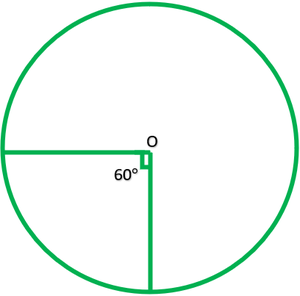### Question 2. Find the area of a quadrant of a circle whose circumference is 22 cm. Quadrant of circle is sector making 90°.

Solution:

Given: circumference of circle=22cm and ∅=90°

To find r=?

2πr=22

=90/360*22/7*7/2*7/2

=77/8cm2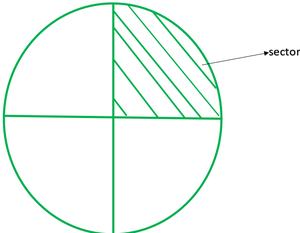### Question 3. The length of the minute hand of a clock is 14 cm. Find the area swept by the minute hand in 5 minutes.

Solution:

Let assume, Minute hand of clock acts as radius of the circle.

Angle rotated by min⁡ (hand in 5minutes)=∅=360/60*5=30°

Area of swept middle hand=

=∅/(360°) *πr2

=30/360*22/7*14*14

=154/3cm2

Area swept by the minute hand in 5min=154/3cm2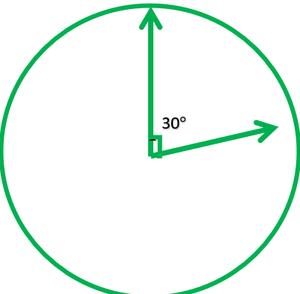### Question 4. A chord of a circle of radius 10 cm subtends a right angle at the centre. Find the area of the corresponding: (i) minor segment (ii) major sector. (Use π = 3.14)

Solution:

Major segment is =360°-90°=270°

(i) Area of minor segment =Area of sector-Area of triangle

=∅/(360°) *πr2-1/2*h*b

=90/306*3.14*10*10-1/2*10*10

=314/4-50

=78.5-50

Area of minor segment =28.5cm2

(ii) Area of major sector=∅/(360°) *πr2

=270/360*3.14*10*10

=3*314/4=235.5cm2

Area of major segment=235.5cm2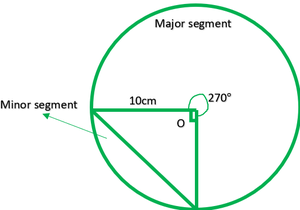### Question 5. In a circle of radius 21 cm, an arc subtends an angle of 60° at the centre. Find:

(i) the length of the arc

(ii) area of the sector formed by the arc

(iii) area of the segment formed by the corresponding chord

Solution:

Length of arc=∅/(360°) *2πr

60/360*2*22/7*21

=22cm

(ii) Area of sector=∅/(360°) *πr2

60/360*22/7*21*21

11*21=231cm2

(iii) Area of segment =Area of sector -Area of triangle

=231-√3/4(side)2

=231-1.73/4*21*21

=231-762.93/4

=231-190.73

=40.27cm2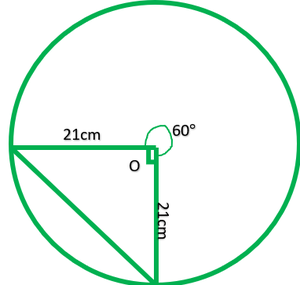### Question 6. A chord of a circle of radius 15 cm subtends an angle of 60° at the centre. Find the areas of the corresponding minor and major segments of the circle. (Use π = 3.14 and √3 = 1.73)

Solution:

∆AOB is isosceles

∴∠A = ∠B

=∠A+∠B+C=180°

=2∠A=180°-60°

=∠A=120°/2

=∠A=60°

Area of minor segment =Area of sector-Area of triangle

=∅/(360°) *πr2-√3/4(side)2

=(60°)/360*3.14*15*15-1.73/4

=706.5/6-389.25/4

=117.75-97.31

=20.44cm2

Area of major segment-Area of circle-Area of minor segment

=πr2-20.44

=3.14*15*15-20.44

=686.06cm2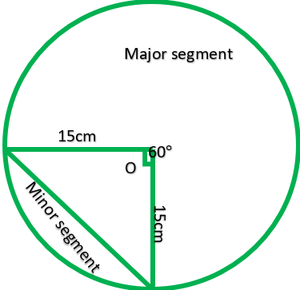### Question 7. A chord of a circle of radius 12 cm subtends an angle of 120° at the centre. Find the area of the corresponding segment of the circle. (Use π = 3.14 and √3 = 1.73)

Solution:

Area of triangle=1/2*base*height

Area of segment=Area of sector -area of triangle

=∅/(360°)*π*r2-1/2(side)2*sin∅

=120°/360°*3.14-1/2(side)2*sin120°∅

=150.72-6*12*sin60°

=150.72-6*12*√3/2

=150.72-36*1.73

=150.72-62.28

=88.44cm2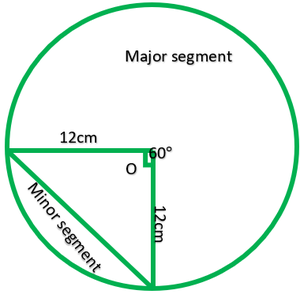### Chapter 12 Areas Related to Circles – Exercise 12.2 | Set 2

Attention reader! Don’t stop learning now. Join the First-Step-to-DSA Course for Class 9 to 12 students , specifically designed to introduce data structures and algorithms to the class 9 to 12 students

My Personal Notes arrow_drop_up